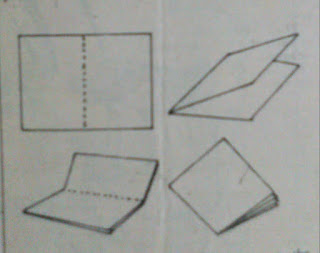# MATHEMATICS 2 by Dr Khin Maung Win (Math)

MATHEMATICS 2 by Dr Khin Maung Win (Math)

FOLDING A PAPER

The Working People’s Daily, 28 June 1987

What’s so special about folding a paper?, you might ask.Nothing special except that you wouldn’t believe it if I tell you that you cannot fold a paper nine times.Nothing like trying.Folding a paper

To do it,take any piece of paper and fold it in half as shown.Then fold it again,in half.That makes two times.Fold it again and go on folding it in half.Generally,it will be almost impossible to fold it nine times.If you do it with any of the daily newspapers or weekly journals, I can tell you that it’s impossible because I have tried it myself.

Why do I find it impossible? Let us see what kind of results a mathematical investigation will yield.

Let us start at the beginning.When you fold a paper once,you get two sheets of paper,one on top of the other. Fold it a second time,and you get four sheets.Fold it a third time and there will be eight sheets.Notice that the number of sheets after each fold is twice the number before.

Now,we construct a table showing the number of times you fold and the number of sheets of paper obtained.Remember that twice or two times is the magic formula.

Number of folds Number of sheets

1 2

2 2.2=4

3 2.2.2=8

4 2.2.2.2 = 16

5 2.2.2.2.2 = 32

6 2.2.2.2.2.2 = 64

7 2.2.2.2.2.2.2 = 128

8 2.2.2.2.2.2.2.2= 256

After folding 8 times, you will have 256 sheets.Now, mathematically you can go on multiplying the results by 2 to obtain

256 x 2 = 512

512 x 2 = 1024

1024 x 2 =2048

But practically , it does not mean that you can go on folding the paper without end.Practice shows that for me it’s impossible to fold it for the ninth time.Why don’t you try ?